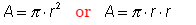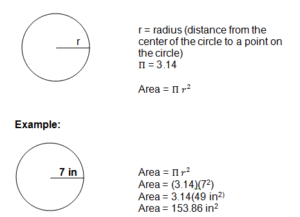# Java Program To Calculate Area Of Circle | 5 Ways

Java program to calculate or to print area of a circle in a simple method. If you were new to java or at the beginning stage then, Check – 500+ simple Java programs for beginners.

The following Java program to print the area of a circle has been written in five simple different ways, static method, using constructor, Interface, inheritance with sample outputs for each program.

1. Using Static Method
2. Using Interface
3. Inheritance
4. Using Constructor
5. Using Method

Also Check : Perimeter of Circle Java

# Below is the online execution tool, for the following program 🙂

## Print Area Of Circle 5 Different Ways With Examples

Below is the program to calculate the AOC in java, if you were new to java coding then we can also share the complete step by steps with detailed explanation on how this java program works, just below this code check it out.

1. Static Method

Output:

As we know that formula in math is :But, if you were written like that, then the system won’t understand, until and unless you assign the value of “PIE” is 22/7.  As usual , you always represent the values in binary numbers.

The system can’t able to understand whether the given number is a number, or given letter is a letter.  All it understood as ” Character ” or just a “ Symbol “. Here goes the complete step by step explanation of the above code and how it works.

Step – 1:

( here ‘import’ is a keyword in java used to get features from inbuilt packages. here we using a package called until it consists of many classes and we using one of the class Scanner to get command over console which is the interface between user and program. )

Step – 2:

( The main function, where the execution of  program start  from here onwards )

Step – 3:

( 1. The scanner is a class used to scan the input data which was given by the user through a console.

so to get access on a console we want to create an object  Syntax:new Scanner(); after creating an object that reference will store in variable ‘s’ )

Step – 4:

( above code is giving instructions for the user to  give the input  for radius)

Step – 5:

( here above instruction is get input in the required format.  first we want to know about  nextDouble() method it takes a token(collection of symbols divide by white space  example:”ABC”,  “DEF” , “GHI”,”10″,”20″)

which is given by  user.when user give 10 as input actually in user perspective  it is number but any number or string  which entered on  console by default  those are  strings,  1o as  string but we want  10 as  number format  for that we have method to convert string to number(Int, Double, Float, Long…..)  some of those method are:

1.nextDouble() ,

2 nextFloat(),

3.nextInt(),

4.nextLong()

5.nextShort()

6.next() or nextString() ).

Step – 6:

Step – 7: System.out.println(“Area of Circle is: ” + area);   ( Once, you entered the radius , the value stored in a particular function ( nextDouble(); ) and read those values with the help of a scanner and display the output for a given value.

A : The major difference is where double can represent the output even after the decimal point , whereas in ” Int ” only the numbers before decimal point will take into consideration.The second method for the above program #sample method – 2, with online compile using command line arguments method :

Here is the sample line for an integer :

let arg=”10″;

int r=Integer.parseInt(args);//r=10;

The purpose of using the “Double ” is , whenever you enter the radius of a particular number like “7” , the answer is 153.86 , numbers after decimal points will also be displayed on the screen. Whereas if you use the “int” , the digits   after the decimal point will be loss.

If you have any doubts related to this program , do comment here. We are glad to help you out.

### 2. Using Interface

There you go another program using the interface with sample outputs.

#### 3. Java Program Using Inheritance

• Another program to print AOC using inheritance with sample example output.
Output:
##### 4. Using Constructor

Using constructor to print AOC with outputs.

Output:

5. Using Method

More Programs:

x

## X Star Pattern Java Program – Patterns

Java program to print X star pattern program – We have written the below print/draw ...